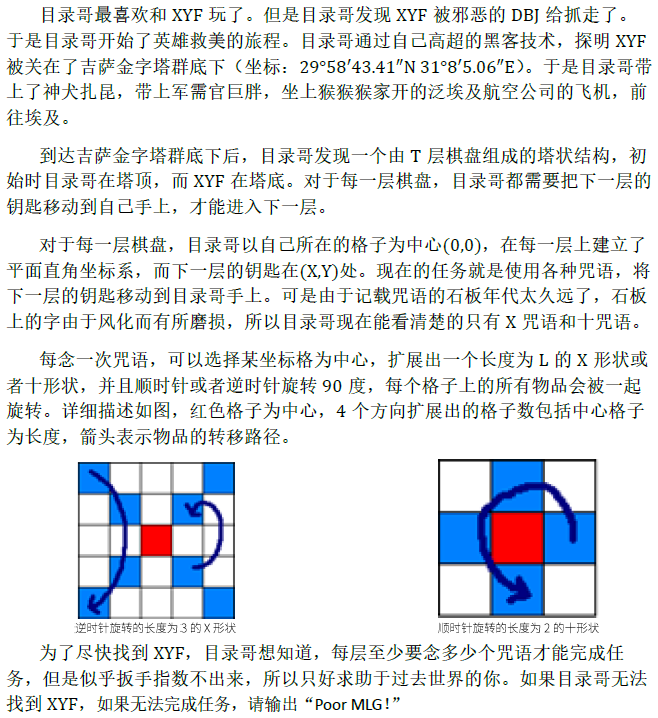# Description# Sample Input

3
12 20 5
14 22 5
0 1 2

# Sample Output

4
5
Poor MLG!

# Solution

a.x>=2l并且y>=2l。此时（x%2l,y%2l）中横纵坐标的其中一个可以少走一些步数，因为我们保证了横纵坐标之差为偶，因此一定可以让x%2l和y%2l的较小值少走一些步数使得两个坐标等于它们的较大值。此时直接用方式二走，t=max(x%2l,y%2l)/l上取整

b.如果其中一个坐标>=2l，另一个<2l。考虑如果大于2l的坐标%2l之后还比<2l的坐标小，肯定能让它少走一点，使得它等于<2l的坐标，这样一定不会更劣。之后就转化到c的情况

c.这里讨论的情况即xy坐标都固定不动（不能少走）的情况。如果两坐标中的较小值>=l，那么先让两坐标减去l，使得两坐标中较小值小于l,注意如果走完之后是奇数坐标就要让两个坐标在退回一步保证变成偶数（即x+1,y+1）。之后剩下的情况即为较小值小于l，那么我们再让两坐标减去两坐标的较小值（至少让一个变为0），剩下的再用一次2l走完（+1步）。

d.t=x不为0则要走一步+y不为0则要走一步+（x,y）为奇数

# Code

#include<cstring>
#include<cstdio>
#include<algorithm>
#include<cstdlib>
#define I int
#define ll long long
#define F(i,a,b) for(register I i=a;i<=b;i++)
#define Fd(i,a,b) for(register I i=a;i>=b;i--)
#define mem(a,b) memset(a,b,sizeof a)
#define N 1010
using namespace std;
I T,x[N],y[N],t[N],q[N],now[N],s[N],ss[N],r[N],l[N],c[N],d[N],e[N],ans[N],bz1,bz2,cnt1,cnt2;
char ch;
void R(I &w){
w=0;ch=getchar();
while(ch<'0'||ch>'9') ch=getchar();
while(ch>='0'&&ch<='9'){w=w*10+ch-'0';ch=getchar();}
}
void in(I x[]){
t=0;
ch=getchar();
while(ch<'0'||ch>'9') ch=getchar();
while(ch>='0'&&ch<='9'){t[++t]=ch-'0';ch=getchar();}
x=t;
F(i,1,x) x[i]=t[x-i+1];
}
void up(I x[]){
F(i,1,x){x[i+1]+=x[i]/10;x[i]%=10;}
while(x[x+1]){
x++;
x[x+1]+=x[x]/10,x[x]%=10;
}
}
mem(t,0);
t=max(a,b);
F(i,1,t) t[i]=a[i]+b[i];
up(t);
}
void dec(I a[],I b[]){
F(i,1,b) a[i]-=b[i];
F(i,1,b){while(a[i]<0) a[i]+=10,a[i+1]--;}
while(a&&!a[a]) a--;
}
void mul(I a[],I val){F(i,1,a) a[i]*=val;up(a);}
I cmp(I a[],I b[]){
if(a>b) return 1;
if(b>a) return 0;
Fd(i,a,1){
if(a[i]>b[i]) return 1;
if(b[i]>a[i]) return 0;
}
return 1;
}
void div(I a[],I b[]){
mem(q,0),mem(r,0);
Fd(i,a,1){
mul(r,10);r+=a[i];up(r);
Fd(j,9,0){
mem(now,0);
F(k,0,b) now[k]=b[k];
mul(now,j);
if(cmp(r,now)){q[i]=j;dec(r,now);break;}
}
}
q=a;
while(q&&!q[q]) q--;
}
I main(){
freopen("movement.in","r",stdin);
freopen("movement.out","w",stdout);
R(T);
while(T--){
mem(x,0),mem(y,0),mem(l,0);
in(x),in(y),in(l);
if(t&1){printf("Poor MLG!\n");continue;}
l--;
F(i,1,l) if(l[i]<0){l[i]+=10,l[i+1]--;}
while(l&&!l[l]) l--;

mem(e,0);
F(i,0,l) e[i]=l[i];
mul(e,2);up(e);
bz1=bz2=1;
div(x,e);
if(cmp(x,r)&&cmp(r,x)) bz1=0;
mem(x,0),mem(s,0);
F(i,0,r) x[i]=r[i];
F(i,0,q) s[i]=q[i];

div(y,e);
if(cmp(y,r)&&cmp(r,y)) bz2=0;
mem(y,0),mem(ss,0);
F(i,0,r) y[i]=r[i];
F(i,0,q) ss[i]=q[i];

if(!bz1&&bz2&&!cmp(y,x)) F(i,0,x) y[i]=x[i];
if(bz1&&!bz2&&!cmp(x,y)) F(i,0,y) x[i]=y[i];
//情况b
mem(ans,0);
F(i,0,t) ans[i]=t[i];
cnt1=cnt2=0;
cnt1=(x>0)+(y>0)+(x&1||y&1);
//情况d
if(bz1&&bz2){
if(x||y){
mem(s,0);
if(cmp(x,y)) F(i,0,x) s[i]=x[i];else F(i,0,y) s[i]=y[i];
//s=max(x,y);
cnt2+=!cmp(l,s)?2:1;
}
//情况a
}
else{
mem(s,0);
if(cmp(x,y)) F(i,0,y) s[i]=y[i];else F(i,0,x) s[i]=x[i];
//s=min(x,y);
if(cmp(s,l)){
dec(x,l);dec(y,l);cnt2++;
if(x&1){x++;y++;up(x),up(y);}
}
mem(s,0);
if(cmp(x,y)) F(i,0,y) s[i]=y[i];else F(i,0,x) s[i]=x[i];
//s=min(x,y);
dec(x,s);dec(y,s);cnt2+=(s>0)+(x>0)+(y>0);
//情况c
}
ans+=min(cnt1,cnt2);
up(ans);
printf("%d",ans[ans]);
Fd(i,ans-1,1) printf("%d",ans[i]);
printf("\n");
}
return 0;
}

12-0112-03
06-222854
06-26580
09-21
08-27